# GMAT Math : Diameter

## Example Questions

### Example Question #1 : Dsq: Calculating The Length Of The Diameter

What is the circumference of a circle?

(1)The diameter of this circle is 10

(2)The area of this circle is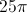BOTH statements TOGETHER are sufficient, but NEITHER statement ALONE is sufficient.

Statement (2) ALONE is sufficient, but statement (1) alone is not sufficient.

Statements (1) and (2) TOGETHER are NOT sufficient.

EACH statement ALONE is sufficient.

Statement (1) ALONE is sufficient, but statement (2) alone is not sufficient.

EACH statement ALONE is sufficient.

Explanation:

The calculation of the circumference is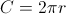. From statement (1) we know that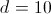. Therefore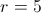, and we can calculate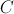using the formula. From statement (2) we know that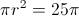. Therefore, and we can calculateusing the formula.

### Example Question #1 : Diameter

Ifandare points on a plane andlies inside the circlewith center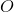and radius 5, doeslie inside circle?

(1) The length of line segment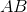is 7.

(2) The length of line segment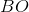is 7.

BOTH statements TOGETHER are sufficient to answer the question, but NEITHER statement ALONE is sufficient.

BOTH statements TOGETHER are not sufficient to answer the question.

Statement 2 ALONE is sufficient to answer the question, but the other statement alone is not sufficient.

EACH statement ALONE is sufficient to answer the question.

Statement 1 ALONE is sufficient to answer the question, but the other statement alone is not sufficient.

Statement 2 ALONE is sufficient to answer the question, but the other statement alone is not sufficient.

Explanation:

(1) The max distance between two points in the circle is twice the length of the

raidus (diameter =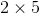=). However,can still be anywhere on the plane

(outside of the circle) as the statement does not indicate otherwise. Therefore, this statement is insuffieicent.

(2) The length of the line segment fromtois greater than the radius of the circle. Thus,must be outside of the circle. This statement is sufficient.

### Example Question #1 : Dsq: Calculating The Length Of The Diameter

Find the diameter of circle B.

I) Circle B has a circumference of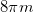.

II) Circle B has an area of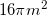.

Neither statement is sufficient to solve the question. More information is needed.

Both statements taken together are sufficient to solve the question.

Statement 2 is sufficient to solve the question, but statement 1 is not sufficient to solve the question.

Statement 1 is sufficient to solve the question, but statement 2 is not sufficient to solve the question.

Each statement alone is enough to solve the question.

Each statement alone is enough to solve the question.

Explanation:

We are given the area and circumference of a circle and asked to find the diameter.

Given the following equations: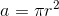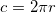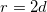We can see that having either area or circumference will allow us to find the radius and in turn the diameter.

Thus, either statement is sufficient by itself.

### Example Question #1 : Dsq: Calculating The Length Of The Diameter

Find the diameter of circle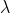I) The area ofofis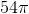.

II) An arc making up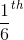ofis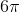.

Either statement alone is sufficient to answer the question.

Statement II is sufficient to answer the question, but Statement I is not sufficient to answer the question.

Both statements together are needed to answer the question.

Statement I is sufficient to answer the question, but Statement II is not sufficient to answer the question.

Either statement alone is sufficient to answer the question.

Explanation:

I) Gives us a portion of the area of the circle. From this we can find the total area and solve for the radius. Then we can double our answer to find the diameter.

II) Gives us a portion of the circumference. From this we can find the total circumference and work our way back to the radius and then the diameter.

### Example Question #72 : Circles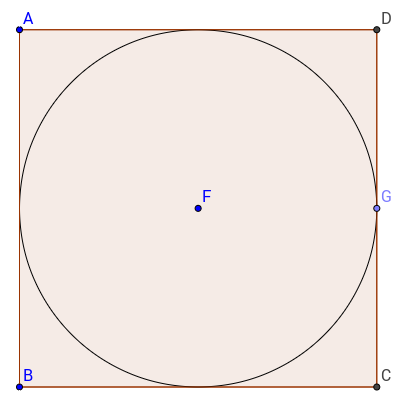The circle of centeris inscribed in square. What is the diameter of the circle?

(1) The ratio of the diameter to the circumference of the circle is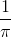.

(2) The area of squareis.

Statement 1 alone is sufficient.

Statments 1 and 2 together are not sufficient.

Both statements together are sufficient.

Each statement alone is sufficient.

Statement 2 alone is sufficient.

Statement 2 alone is sufficient.

Explanation:

To find the diameter of the circle we would need information about the square or about the circle itself.

Statement 1 gives us a ratio of the diameter to the circumference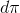of the circle.

If we write the equation it is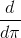or.

Therefore, for all circles, the ratio of the diameter to the circumference will be.

This statement is not helping.

Statement 2 on the other hand gives us the area of the square and therefore allows us to calculate the side of the circle, which is the same as the diameter.

Hence, statement 2 alone is sufficient.

### Example Question #1 : Dsq: Calculating The Ratio Of Diameter And Circumference

Find circle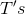ratio of diameter to circumference.

I) The area of circle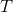is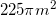.

II) Chord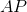passes withinmeter of the center and has a length ofmeters.

Statement I is sufficient to answer the question, but statement II is not sufficient to answer the question.

Both statements are needed to answer the question.

Either statement is sufficient to answer the question.

Statement II is sufficient to answer the question, but statement I is not sufficient to answer the question.

Either statement is sufficient to answer the question.

Explanation:

To find the ratio of diameter to circumference we need our diameter and circumference.

We can use I to find our radius and from there our diameter and circumference.

II can be used to make a triangle with sides of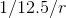which we can then use to find the radius and from there the diameter/circumference.

Either statement is sufficient to answer the question.

### Example Question #7 : Diameter

What is the ratio of the circumference to the diameter of Circle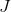?

1.) The radius of the circle is.

2.) The area of the circle is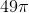Statement 1 is sufficient to solve the question, but Statement 2 is not sufficient to solve the question.

Each statement alone is sufficient to solve the question.

Both statements taken together are sufficient to solve the question.

Neither statement is sufficient to solve the question. More information is needed.

Statement 2 is sufficient to solve the question, but Statement 1 is not sufficient to solve the question.

Each statement alone is sufficient to solve the question.

Explanation:

We are asked to find the ratio of the circumferenceto the diameterof Circleand are given the radius and the area. We also know that.  Taking each statement individually:

1.) The radiusisand we know that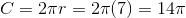. The diameter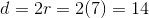. Since we can determine that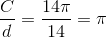,  Statement 1 is sufficient to solve for the ratio by itself.

2.) The areaof the outside circle is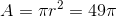, so therefore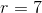. Since we can useto determine both the circumferenceand diameter, Statement 2 is sufficient to solve for the ratio by itself.

### Example Question #621 : Data Sufficiency Questions

Circle A and circle B are given. If the diameter of circle B is, what is the diameter of circle A

1. The circumference of circle A is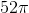.
2. The ratio of the diameters of circle A and circle B is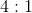, respectively.

Statement 1 alone is sufficient, but statement 2 alone is not sufficient to answer the question.

Statement 2 alone is sufficient, but statement 1 alone is not sufficient to answer the question.

Statements 1 and 2 are not sufficient, and additional data is needed to answer the question.

Both statements taken together are sufficient to answer the question, but neither statement alone is sufficient.

Each statement alone is sufficient to answer the question.

Each statement alone is sufficient to answer the question.

Explanation:

Statement 1: If we know the circumference, we can calculate the diameter.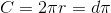If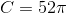then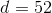Statement 2: We know the diameter of circle B isand that the ratio of the circles. We can set up our proportions and find the diameter: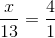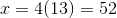### Example Question #71 : Circles

What is the diameter of the circle?

1. The diameter to radius ratio is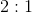.
2. The circumference is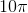.

Statement 1 alone is sufficient, but statement 2 alone is not sufficient to answer the question.

Both statements taken together are sufficient to answer the question, but neither statement alone is sufficient.

Statements 1 and 2 are not sufficient, and additional data is needed to answer the question.

Statement 2 alone is sufficient, but statement 1 alone is not sufficient to answer the question.

Each statement alone is sufficient to answer the question.

Statement 2 alone is sufficient, but statement 1 alone is not sufficient to answer the question.

Explanation:

Statement 1: We're given a ratio (which you should already know) but no values. We need additional information to answer the question.

Statement 2: If we're given the circumference, we can solve for the diameter.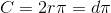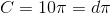which means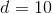Statement 2 alone is sufficient, but statement 1 alone is not sufficient to answer the question.

### Example Question #1 : Diameter

What is the circumference of the circle?

1. The diameter of the circle is.
2. The area of the circle is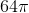.

Each statement alone is sufficient to answer the question.

Statement 2 alone is sufficient, but statement 1 alone is not sufficient to answer the question.

Statements 1 and 2 are not sufficient, and additional data is needed to answer the question.

Both statements taken together are sufficient to answer the question, but neither statement alone is sufficient.

Statement 1 alone is sufficient, but statement 2 alone is not sufficient to answer the question.

Each statement alone is sufficient to answer the question.

Explanation:

Statement 1: We can calculate the circumference using the given diameter.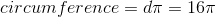Statement 2: To find the circumference, we must first find the radius of the circle using the given area.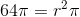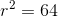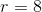We can plug this value into the equation for circumference: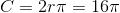Each statement alone is sufficient to answer the question.

### All GMAT Math Resources